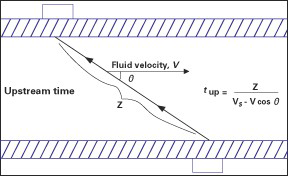# What is transit-time flowmeter?

Transit-time flow measurement technique uses ultrasonic pulse that is projected into and across the pipe.

The transit-time meter’s basic premise is to calculate the time difference (or frequency shift) between downstream flight time and upstream flight time. Then this frequency change can be translated through the pipe into a fluid flow rate.

The figure below shows two transducers attached to a pipe to help explain a type of transit-time design:

V is the average velocity of the fluid, Z is the distance from the upstream to the downstream transducer, and q is the angle between the ultrasonic beamline and the horizontal fluid flow. It is possible to write the time it takes for the ultrasonic signal to travel from the upstream transducer to the downstream transducer.

tdown = Z/(Vs + V cosθ)

where Vs is the velocity of sound through the liquid. The upstream time can be written as:tup = Z/(Vs - V cosθ)

Because it is possible to generate upstream and downstream frequencies in proportion to their respective transit times, we can say:

fdown = 1/tdown

and

fup = 1/tup

Where the downstream and upstream frequencies are represented by fdown and fup. The frequency change can then be specified as:

Δf = fdown - fup = 1/tdown - 1/tup

By substitution, one obtains:

Δf = (Vs + V cosθ)/Z - (Vs - V cosθ)/Z = (2 cosθ/Z)V

Since (2 cosθ/Z) is just a constant, one can write the final equation as:

Δf = kV

with

k = 2 cosθ/Z

That, then, is the basic relationship used by the calculated frequency shift to determine flow velocity. A Reynolds-number adjustment for the velocity profile and programming in the internal pipe diameter can then determine the flow rate.

The correction of the Reynolds-number takes into account the fluid’s behavior as laminar, transitional, or turbulent. Such measurements are made electronically and the overall flow rate or flow can then be shown in the chosen engineering units.

Surprisingly enough, in this instrument, the frequency change is measured independently of Vs. This is an advantage because the line-pressure and temperature variations will not need to be corrected for the variation of Vs.

Advantages of transit-time flow measurement:

• No moving parts

• Zero pressure drop

• Easy installation—transducer

• No process contamination

• Available meters that operate with characteristics of laminar, turbulent or transition flow.

• For remote or field applications, battery-operated units are available

• Sensors for pulsating flows

• For remote or field applications, battery-powered units are available

• Insensitive to changes in liquid temperature, viscosity, density or pressure

1 Like